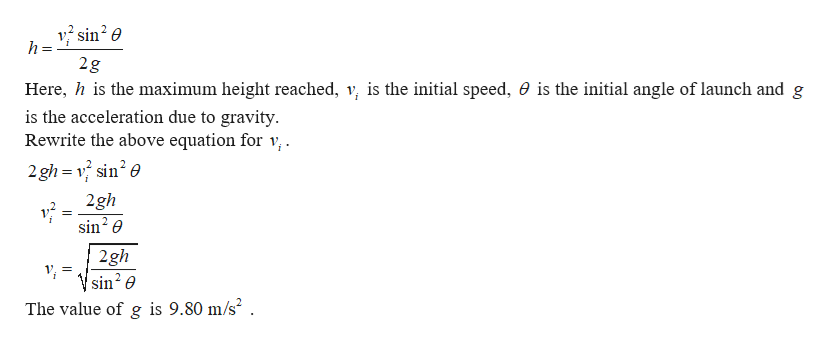# The best leaper in the animal kingdom is the puma, which can jump to a height of 3.7 m when leaving the ground at an angle of 45°. With what speed must the animal leave the ground to reach that height?__ m/s

Question
43 views

The best leaper in the animal kingdom is the puma, which can jump to a height of 3.7 m when leaving the ground at an angle of 45°. With what speed must the animal leave the ground to reach that height?

__ m/s

check_circle

Step 1

The puma will be in a projectile motion. The equation for the maximum height achieved by a projectile is given byhelp_outlineImage Transcriptioncloseh = 2g Here, his the maximum height reached, v is the initial speed, 0 is the initial angle of launch and g is the acceleration due to gravity Rewrite the above equation for v, 2gh=v sin2 e 2gh sin e 2gh sin e The value of g is 9.80 m/s2 . fullscreen
Step 2

Substitute the numerical values...

### Want to see the full answer?

See Solution

#### Want to see this answer and more?

Solutions are written by subject experts who are available 24/7. Questions are typically answered within 1 hour.*

See Solution
*Response times may vary by subject and question.
Tagged in

### Physics